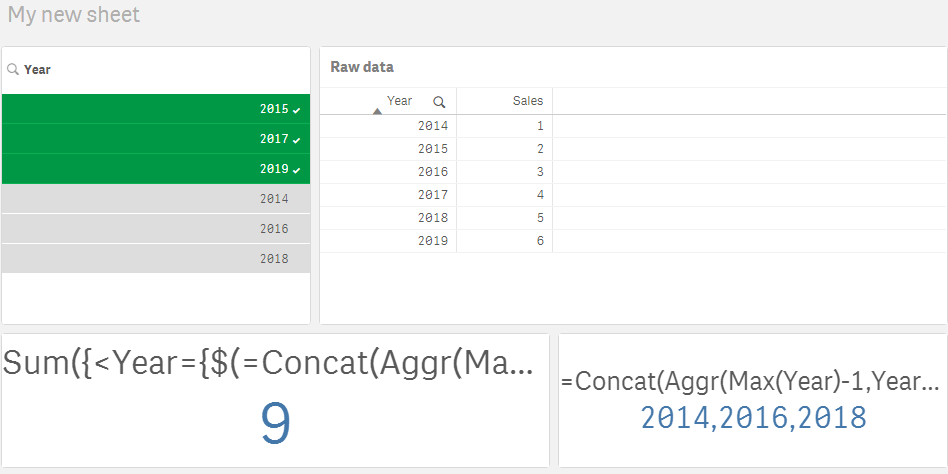# New to Qlik Sense

If you’re new to Qlik Sense, start with this Discussion Board and get up-to-speed quickly.

Announcements
Our May 2021 end-to-end product release from Data Integration to Data Analytics is out! READ DETAILS
cancel
Showing results for
Search instead for
Did you mean:Partner

## Retrieving years minus 1

I try to make an expression to retrieve values based on selected years, but where the year value are subtracted with one year. For example when selecting the year 2019, I want values for 2018. When I select 2017, 2018, 2019 I want values for 2016,2017,2018.

I have made this expression:

Sum({<Year={\$(=GetFieldSelections(Year)-1)}>}Sales)

It works fine when selecting one year. For example, if I select 2019 it gives me 2018.

But when I select several years (2019, 2018, 2017) it ends in nothing.

Labels (1)
• ### Set Analysis Expression

2 Solutions

Accepted SolutionsMVP

Another option is to use this

``Sum({<Year = {">=\$(=Min(Year)-1)<=\$(=Max(Year)-1)"}>} Sales)``Creator III

@jelindbe  I figured out the correct expression now. It's much simpler than I initially thought 😀.

Concat(Aggr(...))  can be used in the set modifier to form the search string:

``=Sum({<Year={\$(=Concat(Aggr(Max(Year)-1,Year),','))}>}Sales)``

This one works with both range and discrete selections:BR,

Vu Nguyen

6 RepliesCreator III

Hi,

The following expression works, but only when you select a connected range of year (for example 2017,2018,2019)

``=Sum({<Year={">=\$(=Left(GetFieldSelections(Year),4)-1) <=\$(=Right(GetFieldSelections(Year),4)-1)"}>}Sales)``

The expression inside Set Analysis generates a search string like ">=2016 <=2018", which can be applied  as a set modifier.

This doesn't work if you also need to select discrete years (for example 2016, 2017, 2019) because the generated search string would be ">=2015 <=2018", which means 4 years 2015,2016,2017,2018.

Hope this helps,

BR,

Vu NguyenMVP

Another option is to use this

``Sum({<Year = {">=\$(=Min(Year)-1)<=\$(=Max(Year)-1)"}>} Sales)``Creator III

Yes of course, I over-complicated the solution. This is a better one 🙂Partner
Author

Thank you!Creator III

@jelindbe  I figured out the correct expression now. It's much simpler than I initially thought 😀.

Concat(Aggr(...))  can be used in the set modifier to form the search string:

``=Sum({<Year={\$(=Concat(Aggr(Max(Year)-1,Year),','))}>}Sales)``

This one works with both range and discrete selections:BR,

Vu NguyenPartner
Author

You are right! That one worked.

Perhaps you could come up with a nice solution for my label.

So far I have this solution: 'my variable description ' &(Min(Year)-1) &'-' &(Max(Year)-1). This was OK for range selections, but is not good for discrete selections.

All the best and good weekend!